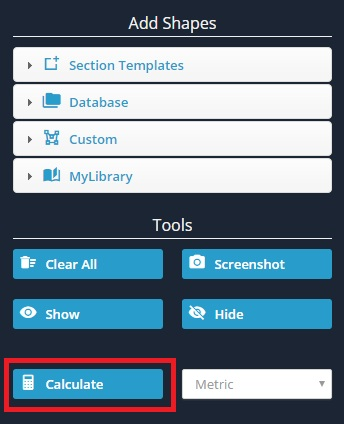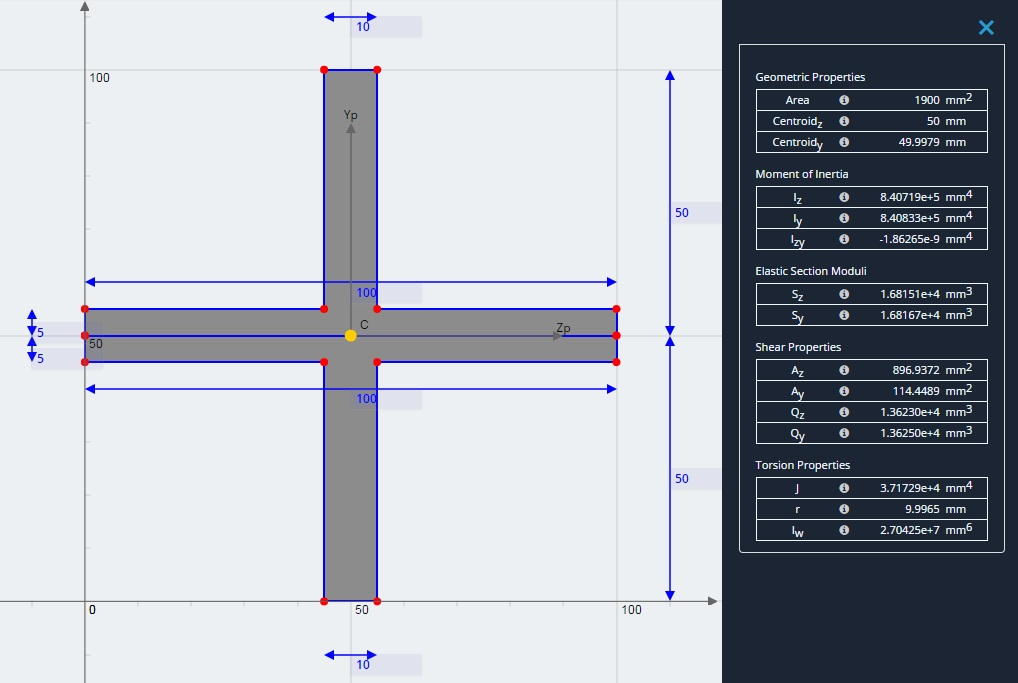Documentation [Deprecated]
SkyCiv Documentation

Getting Started

Section Builder
Operations
Built-Up and Composite Sections
Custom Shapes

Member Design
General
Member Design Modules
Code Verification

RC Design
General
Code Verification

# Standard Results

The main purpose of the Section Builder is to calculate the various section properties of your section. The standard results refer to the results of a section when ignoring material property differences.

When you've finished building your section, simply click 'Calculate' to determine the section properties. Note that the short wait time associated with each calculate is due to the Finite Element and Meshing that occurs in the background in order to solve for torsion constant, torsion radius, and warping constant. By using a FEA method to calculate these properties, a higher accuracy is achieved. Less robust programs only use approximate equations on the standard sections for these properties and fail to solve when a non standard section (such as the cross beam below) is entered.The results are generally broken down into 4 main categories.

• Geometric Properties
• Area
• Centroid (Z)
• Centroid (Y)
• Bending Properties
• Moment of Inertia
• Iz
• Iy
• Izy
• Elastic Section Moduli
• Sz
• Sy
• Shear Properties
• Shear Area about Z axis (Az)
• Shear Area about Y axis (Ay)
• Static Moment of Area about the Z neutral axis (Qz)
• Static Moment of Area about the Y neutral axis (Qy)
• Torsion Properties
• Torsion Constant (J)